# EP1901510A1 - Peak-to-average-power-ratio reduction in a multicarrier system - Google Patents

## Info

Publication number
EP1901510A1
EP1901510A1 EP20060019360 EP06019360A EP1901510A1 EP 1901510 A1 EP1901510 A1 EP 1901510A1 EP 20060019360 EP20060019360 EP 20060019360 EP 06019360 A EP06019360 A EP 06019360A EP 1901510 A1 EP1901510 A1 EP 1901510A1
Authority
EP
European Patent Office
Prior art keywords
signal
evaluation
input signal
providing
power
Prior art date
Legal status (The legal status is an assumption and is not a legal conclusion. Google has not performed a legal analysis and makes no representation as to the accuracy of the status listed.)
Withdrawn
Application number
EP20060019360
Other languages
German (de)
French (fr)
Inventor
Ivan Cosovic
Current Assignee (The listed assignees may be inaccurate. Google has not performed a legal analysis and makes no representation or warranty as to the accuracy of the list.)
NTT Docomo Inc
Original Assignee
NTT Docomo Inc
Priority date (The priority date is an assumption and is not a legal conclusion. Google has not performed a legal analysis and makes no representation as to the accuracy of the date listed.)
Filing date
Publication date
Application filed by NTT Docomo Inc filed Critical NTT Docomo Inc
Priority to EP20060019360 priority Critical patent/EP1901510A1/en
Publication of EP1901510A1 publication Critical patent/EP1901510A1/en
Withdrawn legal-status Critical Current

## Images

•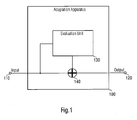•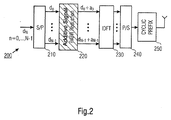•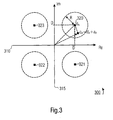•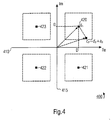•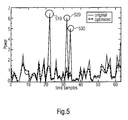•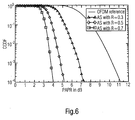•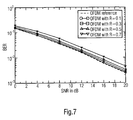•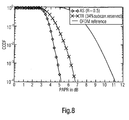## Classifications

• HELECTRICITY
• H04ELECTRIC COMMUNICATION TECHNIQUE
• H04LTRANSMISSION OF DIGITAL INFORMATION, e.g. TELEGRAPHIC COMMUNICATION
• H04L27/00Modulated-carrier systems
• H04L27/26Systems using multi-frequency codes
• H04L27/2601Multicarrier modulation systems
• H04L27/2614Peak power aspects
• HELECTRICITY
• H04ELECTRIC COMMUNICATION TECHNIQUE
• H04LTRANSMISSION OF DIGITAL INFORMATION, e.g. TELEGRAPHIC COMMUNICATION
• H04L27/00Modulated-carrier systems
• H04L27/32Carrier systems characterised by combinations of two or more of the types covered by groups H04L27/02, H04L27/10, H04L27/18 or H04L27/26
• H04L27/34Amplitude- and phase-modulated carrier systems, e.g. quadrature-amplitude modulated carrier systems
• H04L27/3405Modifications of the signal space to increase the efficiency of transmission, e.g. reduction of the bit error rate, bandwidth, or average power
• H04L27/3411Modifications of the signal space to increase the efficiency of transmission, e.g. reduction of the bit error rate, bandwidth, or average power reducing the peak to average power ratio or the mean power of the constellation; Arrangements for increasing the shape gain of a signal set

## Abstract

An adaptation apparatus (100) for adapting an input signal, the input signal being digital in the frequency domain and having a peak-to-average-power-ratio in the time domain. The adaptation apparatus (100) comprises an evaluation unit (130) for evaluating the input signal and for providing an evaluation signal based on an optimization criterion. The adaptation apparatus (100) further comprises a summing unit (140) for adding the evaluation signal to the input signal in the frequency domain and for providing an output signal in the frequency domain having a lower peak-to-average-power-ratio in the time domain than the signal in the time domain.

## Description

• The present invention relates to the field of peak-to-average-power-ratio reduction in wireless communication systems, where low peak-to-average-power-ratios are desired in order to take advantage of the linear characteristics of power amplifiers and in order to avoid inter-modulation products caused by non linear power amplifier characteristics. More specifically, the present invention relates to peak-to-average-power-ratio reduction as required in future mobile communication systems, as for example in orthogonal frequency division multiplexing (OFDM) systems or generally systems, which are based on multi-carrier (MC) modulation.
• A high peak-to-average-power-ratio of a transmit signal is one of the greatest problems of multi-carrier systems, for example, orthogonal frequency division multiplexing (OFDM) or discrete multi-tone (DMT) systems. High peak values drive non-linear power amplifiers during operation in non-linear regions of their amplifying characteristics. Amplifiers being operated in non-linear regions of their characteristics cause inter-modulation products, modulations among sub carriers and out of band radiation. To prevent these effects a power amplifier must be operated in the linear region of its characteristics introducing large input back-offs for the case of higher peaks being present. The problem of higher peak-to-average-power ratios can, in some applications, even compensate or annul the benefits of multi-carrier modulations. Hence, the reduction of the peak-to-average-power-ratio is an essential topic in multi-carrier systems.
• A number of techniques has been proposed to deal with the problem of high peak-to-average-power ratios in orthogonal frequency division multiplexing. The following list provides a summary of the state of the art concepts:
• A comprehensive overview of the above-mentioned techniques including some further references can be found in, S. Hee Han and J. Hong Lee, "An Overview of Peak-to-Average Power Ratio Reduction Techniques for Multi-carrier Transmission", IEEE Wireless Communications, April 2005, pp. 56-65.
• One of the most promising solutions is the tone reservation method. In this method, several sub-carriers are reserved for transmission of peak-to-average-power-ratio reduction tones. The peak-to-average-power-ratio reduction tones are determined so as to minimize the peak-to-average-power-ratio of the original transmission signal. Although the corresponding peak-to-average-power-ratio reduction results are promising, this method suffers from loss in spectral efficiency due to the reservation of exclusive tones for the purposes of peak-to-average-power-ratio reduction. In addition, power efficiency is reduced as part of the transmit power is invested not into data sub-carriers, but on peak-to-average-power-ratio reduction sub-carriers. The tone reservation method is currently under consideration by the third generation partnership project (3GPP) within their technical specification group radio access network as one of the physical layer aspects for evolved Universal Terrestrial Radio Access (UTRA), cf. 3rd Generation Partnership Project (3GPP), "Physical layer aspect for evolved Universal Terrestrial Radio Access (UTRA)", June 2006.
• It is the object of the present invention to provide a new concept for peak-to-average-power-ratio reduction for signals in wireless communication systems, especially multi-carrier systems as currently under consideration for future wireless communication systems, as for example orthogonal frequency division multiplexing.
• This objective is achieved by an adaptation apparatus according to claim 1 and a method for adapting an input signal according to claim 12.
• The objective is achieved by an adaptation apparatus for adapting an input signal, the input signal being digital in the frequency domain and having a peak-to-average-power-ratio in the time domain, the adaptation apparatus comprising an evolution unit for evaluating the input signal and for providing an evaluation signal based on an optimization criterion. The adaptation apparatus further comprises a summing unit for adding the evaluation signal to the input signal in the frequency domain and for providing an output signal in the frequency domain having a lower peak-to-average-power-ratio in the time domain than the input signal in the time domain.
• The objective is achieved by a method for adapting an input signal, the input signal being digital in the frequency domain and having a peak-to-average-power-ratio in the time domain, comprising the step of evaluating the input signal and for providing an evaluation signal based on an optimization criterion. The method further comprises the step of adding the evaluation signal to the input signal in the frequency domain and the step of providing an output signal in the frequency domain having a lower peak-to-average-power-ratio in the time domain than the input signal in the time domain.
• The present invention is based on the finding that an optimization algorithm can be used to find an evaluation signal, which is added to the input signal, e.g. for orthogonal frequency division multiplexing an addition of a complex valued sequence to the original transmit data sequence is applied in a way that the peak-to-average-power-ratio is minimized. The additive complex valued sequence could for example be determined using an efficient convex optimization algorithm, which includes constraints on transmission power and bit error performance.
• The present invention provides the advantage that it requires only additional signal processing at the transmitter whereas the receiver remains unchanged. The inventive apparatus and methods outperform other schemes for peak-to-average-power-ratio reduction and can be applied to basically all orthogonal frequency division multiplexing systems, as for example WLAN, DVB-T, 4G systems, etc.
• Embodiments of the present invention will be detailed using the figures attached, in which:
• Fig. 1
shows a diagram of an embodiment of the inventive adaptation apparatus;
Fig. 2
shows a block diagram of an orthogonal frequency division multiplexing system, wherein an embodiment of an inventive adaptation apparatus is integrated;
Fig. 3
shows an exemplified constellation diagram for quadrature phase shift keying (QPSK) modulation with circular value range regions for the additive signal;
Fig. 4
shows an exemplified constellation diagram for QPSK modulation with rectangular value range regions for the additive signal;
Fig. 5
shows two exemplified time domain signals, before and after adding an inventive peak-to-average-power-ratio reduction signal;
Fig. 6
shows the complementary cumulative distribution function of an exemplified peak-to-average-power-ratio for a conventional OFDM system, and for the proposed additive signal method for different values of radius R;
Fig. 7
shows bit-error-ratios versus signal-to-noise-ratios for an OFDM system applying the inventive method, for different radii R, for N=64 sub-carriers, and assuming a Rayleigh fading channel with QPSK modulation; and
Fig. 8
shows a comparison of an exemplified complementary accumulated distribution function of peak-to-average-power ratios for the inventive additive signal method compared to the state of the art tone reservation method.
• Fig. 1 shows an embodiment of an adaptation apparatus 100 with a connector 110 for an input signal and a connector 120 for an output signal. In the embodiment displayed in Fig. 1 the adaptation apparatus 100 further comprises an evaluation unit 130 and a summation entity 140. The adaptation apparatus 100 is for adapting an input signal, the input signal being digital in the frequency domain and having a peak-to-average-power-ratio in time domain. The input signal could for example be a signal from a WLAN system, a DVB-T system, or a 4G system. Basically, the input signal can be any multi-carrier signal in the frequency domain.
• The adaptation apparatus 100 further comprises the evaluation unit 130 for evaluating the input signal and for providing an evaluation signal based on an optimization criterion. The optimization criterion can comprise several constraints regarding the evaluation signal as for example a power constraint or also a constraint on a bit error rate.
• The adaptation apparatus 100 further comprises a summing unit 140 for adding the evaluation signal to the input signal in the frequency domain and for providing the output signal in the frequency domain having a lower peak-to-average-power-ratio in the time domain than the input signal in the time domain.
• The proposed technique adds signal-processing units, i.e. the evaluation unit 130 and the summing unit 140 only at the transmitter, whereas a potential receiver remains unchanged. The evaluation signal as a peak-to-average-power ratio-reduction signal is added to a transmission signal and it therewith causes some degradation in the system performance, as the transmission symbols do not coincide with the original symbol constellation any more. However, by introducing proper constraints into the design of the peak-to-average-power-ratio reduction signal degradation can be easily controlled and limited.
• In comparison to the tone reservation method, the proposed method exhibits a number of advantages. Assuming the same bit-error-ratio versus signal-to-noise-ratio working point, the proposed method exhibits a lower peak-to-average-power ratio. This is due to the fact that all sub-carriers are exploited for peak-to-average-power-ratio suppression and thus more degrees of freedom are available to find a solution that minimizes the peak-to-average-power-ratio than in the case of the tone reservation method for example.
• The proposed method further provides the advantage that no reservations of special sub-carriers for purposes of peak-to-average-power-ratio reduction are required and it does thus not reduce the spectral efficiency as for example the tone reservation method.
• Fig. 2 illustrates an orthogonal frequency division multiplexing transmitter 200. The transmitter comprises a serial-to-parallel converter 210, an additive signal entity 220, which is an embodiment of the inventive adaptation apparatus, an inverse discrete Fourier transformation entity 230, a parallel-to-serial converter 240, and a cyclic prefix addition entity 250. An orthogonal frequency division multiplexing system with a total of N sub-carriers is considered. According to the illustration in Fig. 2, the input bits are simply mapped applying phase shift keying or quadrature amplitude modulation and N complex valued data symbols dn, n = 0,1..., N-1, are generated. These symbols are serial-to-parallel converted using the serial-to-parallel converter 210. The output of the serial-to-parallel converter 210 is an N element data symbol array d=(d0, d1,...dN-1)T, where (.)T denotes the transposition. The array d is fed into the peak-to-average-power-ratio reduction unit 220, which is an embodiment of the inventive adaptation apparatus. The output of the additive signal peak-to-average-power-ratio reduction unit 220 is another N element symbol array c = (c0, c1..., cN-1)T. In this embodiment of the present invention, the peak-to-average-power-ratio reduction unit 220 adds to each symbol dn a complex valued signal an. Therefore, the entries of c, i.e. the output of the peak-to-average-power-ratio reduction unit 220, are given by $c n = d n + a n , n = 0 , 1 , … , N - 1 .$
• The complex values an, n = 0,1...N-1, are chosen according to an optimization algorithm by which the peak-to-average-power-ratio of the transmission signal is optimized, and in one embodiment of the present invention the peak-to-average-power-ratio of the transmission signal is minimized. Finally, the vector c is modulated on N sub-carriers using the inverse discrete Fourier transform entity 230. After that, a parallel-to-serial conversion is performed using the parallel-to-serial converter 240, and a guard interval of length TΔ that exceeds the delay spread of the multi-path channel is added as cyclic prefix.
• In the following, it will be described how the additive array $a = a 0 ⁢ a 1 … a N - 1 T , a ∈ C N ,$ is designed according to an optimisation criterion by which the peak-to-average-power-ratio is improved, respectively, in one embodiment of the present invention even minimized. The peak-to-average-power-ratio (PAPR) of an OFDM symbol is defined as $PAPR = max k = 0 , 1 , … N - 1 ⁢ x k 2 1 N ⁢ ‖ x ‖ 2$
where x = (x0, x1,..., xN-1)T is the transmitted time domain vector obtained after the inverse discrete Fourier transformation of the transmit symbol vector c = d+a. In particular, $x = Fc = F ⁢ d + a$
where F is the N x N matrix with entries $F m , p = 1 N ⁢ e j ⁢ 2 ⁢ πmp / N m = 0 , 1 , … , N - 1 , p = 0 , 1 , … , N - 1 .$
• The factor $1 N$ in (3) is a scale factor inserted so that the minimum peak-to-average-power-ratio equals 0 dB. To minimize the peak-to-average-power-ratio of the transmission signal x a vector a has to be determined by solving the following optimization problem $a = min a ˜ max k = 0 , 1 , … N - 1 ⁢ F k ⁢ d + a ˜ 2 1 N ⁢ ‖ F ⁢ d + a ˜ ‖ 2$
where ã is a trial value of a and F k is the kth row of matrix F.
• In one embodiment of the present invention, the vector a has two constraints. The first constraint ensures that the sequence c does not invest more power into the transmission than the original sequence d, i.e. $‖ d + a ˜ ‖ 2 ≤ ‖ d ‖ 2 .$
• The second constrain inherently controls the bit error rate (BER) performance and ensures that the elements of a are between pre-defined limits, i.e., $a n ≤ R , n = 0 , 1 , … , N - 1 .$
• The effect of the constraint given in equation (8) on the element cn is illustrated in Fig. 3. Fig. 3 shows a constellation diagram 300 for quadrature phase shift keying (QPSK) modulation. Fig. 3 depicts the complex plane with a real axis 310 and an imaginary axis 315. Furthermore, Fig. 3 shows four modulation symbols 320-323, which all have the same magnitude and are ordered symmetrically to the origin. Taking into account the constraints given in equation (8), one can determine circular regions centered in the modulation symbols 320-323 themselves with the radius of R, wherein the manipulated symbols cn may happen to occur. As it can be seen from Fig. 3 with the constraint given in equation (8) it is allowed that elements cn lie only within the circular regions of radius R around the original points dn. Owing to the fact that the resulting points cn do not coincide with the original points, the bit-error-rate performance is influenced. It can be easily seen from Fig. 3, that when R increases, obviously, it can be expected that the bit-error-ratio also increases as the new points cn can lie closer to the neighbouring decision regions. On the other hand, when R increases, the constraint given in equation (8) becomes looser enabling to find a better solution to the optimization problem given in equation (6).
• Alternatively, instead of defining circular areas as with the constraint given in equation (8), areas with other shapes are also conceivable. For example, rectangular areas in which the manipulated points or symbols cn are allowed to lie are illustrated in Fig. 4. Fig. 4 shows another constellation diagram 400 with a real axis 410, an imaginary axis 415, and four modulation symbols 420-423. Fig. 4 shows the four rectangular areas in which the points cn are allowed to lie as mentioned above.
• The optimization problem given in equation (6), together with the constraints given in equations (7) and (8), provides a convex optimization problem of the variables an, n=0,1...N-1. Convex optimization has been studied extensively and there are very effective and reliable numerical methods to solve this optimization problem, for example, the interior point method as it is described for example in S. Boyd, Convex Optimization, Cambridge University Press, 2004.
• This convex optimization problem has N unknowns and N+1 constraints. The interior point methods can find a solution for such problems in a number of iterations that is almost always in a range between 10 and 100. For each iteration, the complexity is equal to O(N2(N+1)). In this embodiment of the present invention a particular interior point method, the barrier method is applied. This is an iterative method that transforms the optimization problem with constraints in an unconstrained optimization problem. The resulting unconstrained optimization problem can be solved, for example applying the gradient method.
• In details, the function to be minimized is defined as objective function. In this embodiment of the present invention the objective function is the peak-to-average-power-ratio as given in equation (6). The first step is to transform the original inequality constraint problem in an equivalent unconstrained problem. To do this, the inequality constraints of equations (7) and (8) are added to the objective function and the modified objective function is obtained $max k = 0 , 1 , … N - 1 ⁢ F k ⁢ d + a ˜ 2 1 N ⁢ ‖ F ⁢ d + a ˜ ‖ 2 + I_ ‖ d + a ˜ ‖ 2 - ‖ d ‖ 2 + ∑ i = 1 N - 1 I_ a ˜ i - R$
where I_ is the indicator function defined as $I_ u = { 0 u ≤ 0 ∞ u > 0.$
• The basic idea of the barrier method is to approximate the indicator function given in equation (10) by the function $I ^ _ u = - 1 / t ⁢ log - u$
where t>0 is a parameter that determines the accuracy of the approximation. As t increases, the approximation becomes more accurate. Substituting _ for I_ in equation (9) multiplying (9) by t, it is obtained $t max k = 0 , 1 , … N - 1 ⁢ F k ⁢ d + a ˜ 2 1 N ⁢ ‖ F ⁢ d + a ˜ ‖ 2 - log ⁢ ‖ d ‖ 2 - ‖ d + a ˜ ‖ 2 - ∑ i = 1 N - 1 log ⁢ R - a ˜ i .$
• The new objective function as given in equation (12) can be minimized using for example a gradient method.
• A possible drawback of the embodiment of the present invention, which is described above and which is based on the addition of the signal is a degradation in the bit-error-ratio versus the signal-to-noise-ratio performance as, due to the addition of the complex values an, the resulting points cn do not coincide with the original points dn. The bit-error-ratio degradation is analyzed by Monte-Carlo simulations, which are described in the following.
• Fig. 5 shows a plot of two OFDM transmission symbols, one in its original version depicted as a straight line and one which was optimized according to the algorithm described above, depicted by a dotted line. Quadrature phase shift keying with Gray mapping modulation is applied and channel coding is not considered. The number of sub-carriers is set to N = 64. The radius R is set to 0.5 in Fig. 5. Fig. 5 shows a plot of the power of a typical time domain signal before and after the optimization. The benefits of the inventive methods are clearly visible. Looking at the areas 510, 520, and 530, which are marked in Fig. 5, it can clearly be seen how the peak values are reduced, and therewith the peak-to-average-power ratio.
• Fig. 6 presents the results in terms of a complementary cumulative distribution function (CCDF). For the simulations, for which the results are displayed in Fig. 6, the radius R is kept variable. The peak-to-average-power-ratio reduction is averaged over a 100,000 randomly chosen data sequences. The simulation results are given for different radii R. The diagram shown in Fig. 6 shows the complementary cumulative distribution function versus the peak-to-average-power-ratio in dB. Already for a radius of R = 0.5, a remarkable average reduction of more than 6 dB is achieved, when considering a ratio of 10-3 for the CCDF. A further increase of the radius R enables even better suppression. The reason for this lies in the fact that as the radius R grows the constraint from equation (8) becomes looser, therefore allowing more degrees of freedom to find a solution of equation (9).
• The assessment of the Figs. 6 and 7 reveals that there are two counter acting effects caused by the radius R. Enlarging this radius improves the peak-to-average-power-ratio reduction, but simultaneously leads to a further loss in the signal-to-noise-ratio performance. Therefore, there is a trade-off between the additional peak-to-average-power reduction obtained by enlarging the radius R and the increased loss in signal-to-noise-ratio performance. Setting R = 0.5 seems to be a good compromise.
• Fig. 8 shows a comparison of the inventive method of the additive signal with the tone reservation method. For the inventive method of the additive signal a radius of R = 0.5 was assumed and for the tone reservation method 22 out of the 64 sub-carriers were reserved, which leads to a loss of around 34 % in spectral efficiency. That set-up leads to approximately the same bit-error-ratio versus signal-to-noise-ratio performance. As it can be seen from Fig. 8, the inventive method of the additive signal exhibits much lower peak-to-average-power ratio. In addition, the inventive approach has higher spectral efficiency as it does not require reservation of the spectral sub-carriers as the tone reservation method does.
• The present invention provides the advantage that the peak-to-average-power-ratio especially in OFDM systems and multi-carrier systems can be efficiently reduced, as it was shown utilizing numerical results which have been presented in the Figs. 5 to 8. Furthermore, the present invention provides the advantage that it can be applied in many systems as for example WLAN, DVB-T, and 4G systems. Comparing the inventive methods to the state of the art approaches, the present invention further provides the advantage that the spectral efficiency of a transmission system can be kept higher while achieving a lower peak-to-average-power-ratio at the same time.
• Depending on certain implementation requirements of the inventive method, the inventive method can be implemented in hardware or in software. The implementation can be performed using a digital storage medium, in particular a disk, a DVD, or a CD having an electronically readable control signal stored thereon, which cooperate with a programmable computer system such that the inventive methods are performed. Generally, the present invention is therefore, a computer program product with a program code for a machine-readable carrier, the program code being operative for performing the inventive methods when the computer program runs on a computer. In other words, the inventive methods are therefore, a computer program having a program code for performing at least one of the inventive methods when the computer program runs on a computer.
• List of References
• 100
110
Input connector
120
Output connector
130
Evaluation Unit
140
Summing Unit
200
OFDM transmitter
210
Serial-to-parallel converter
220
230
Inverse discrete Fourier transform entity
240
Parallel-to-serial converter
250
300
Constellation Diagram
310
Real Axis
315
Imaginary Axis
320-323
Modulation symbols
400
Constellation Diagram
410
Real Axis
415
Imaginary Axis
420-423
Modulation symbols
510
Peak in original OFDM symbol
520
Peak in original OFDM symbol
530
Peak in original OFDM symbol

## Claims (13)

1. An adaptation apparatus (100) for adapting an input signal, the input signal being digital in the frequency domain and having a peak-to-average-power-ratio in the time domain, the adaptation apparatus (100) comprising:
an evaluation unit (130) for evaluating the input signal and for providing an evaluation signal based on an optimization criterion; and
a summing unit (140) for adding the evaluation signal to the input signal in the frequency domain and for providing an output signal in the frequency domain having a lower peak-to-average-power-ratio in the time domain than the input signal in the time domain.
2. Adaptation apparatus (100) of claim 1, wherein the input signal is a multicarrier signal, the evaluation unit (130) is adapted for evaluating each of the multicarrier components of the input signal for providing a multicarrier evaluation signal with a component for each multicarrier, and the summing unit (140) is adapted for adding the multicarrier components of the multicarrier evaluation signal and the input signal for providing an output signal with a component for each multicarrier.
3. Adaptation apparatus (100) of claim 2, wherein the input signal is an orthogonal frequency division multiplexing or a discrete multitone signal with a plurality of subcarriers, the evaluation unit (130) is adapted for evaluating each of the subcarriers of the input signal for providing a subcarrier evaluation signal with a component for each subcarrier, and the summing unit (140) is adapted for adding the subcarrier components of the subcarrier evaluation signal and the input signal for providing an output signal with a component for each subcarrier.
4. Adaptation apparatus (100) of one of the claims 1-3, wherein the input signal comprises a complex representation of a base band signal;
the evaluation unit (130) is adapted for providing a complex evaluation signal; and
the summing unit (140) is adapted for adding the complex evaluation signal to the input signal.
5. Adaptation apparatus (100), of one of the claims 1-4, wherein the input signal comprises a complex representation of modulation symbols of a phase shift keying or a quadrature amplitude modulation symbol alphabet and the summing unit (140) is adapted for adding the evaluation signal to the complex representation of modulation symbols of a phase shift keying or a quadrature amplitude modulation symbol alphabet.
6. Adaptation apparatus (100) of one of the claims 1-5, wherein the evaluation unit (130) is adapted for providing the evaluation signal based on a convex optimization algorithm.
7. Adaptation apparatus (100) of one of the claims 1-6, wherein the evaluation unit (130) is adapted for providing the evaluation signal according to an optimization criterion including constraints on a transmission power or a bit-error-rate performance.
8. Adaptation apparatus (100) of one of the claims 1-7, wherein the evaluation unit (130) is adapted for providing the evaluation signal according to an optimization criterion comprising a sub-criterion such that the power of an output signal is less or equal to a power of the input signal.
9. Adaptation apparatus (100) of one of the claims 1-8, wherein the evaluation unit (130) is adapted for providing the evaluation signal according to an optimization criterion comprising a sub-criterion such that the value range of the symbols of the evaluation signals are constrained.
10. Adaptation apparatus (100) of one of the claims 1-9, wherein the evaluation unit (130) is adapted for providing the evaluation signal according to an optimization criterion comprising a sub-criterion such that the magnitudes of the symbols of the evaluation signals are constrained.
11. Adaptation apparatus (100) of one of the claims 1-10, wherein the evaluation unit (130) is adapted for providing the evaluation signal based on an interior point method, a barrier method or a gradient method.
12. Method for adapting an input signal, the input signal being digital in the frequency domain and having a peak-to-average-ratio in the time domain, comprising the steps of:
evaluating the input signal and providing an evaluation signal based on an optimization criterion;
adding the evaluation signal to the input signal in the frequency domain; and
providing an output signal in the frequency domain having a lower peak-to-average-power-ratio in the time domain than the input signal in the time domain.
13. Computer program having a program code for performing the method of claim 12, when a program code runs on a computer.
EP20060019360 2006-09-15 2006-09-15 Peak-to-average-power-ratio reduction in a multicarrier system Withdrawn EP1901510A1 (en)

## Priority Applications (1)

Application Number Priority Date Filing Date Title
EP20060019360 EP1901510A1 (en) 2006-09-15 2006-09-15 Peak-to-average-power-ratio reduction in a multicarrier system

## Applications Claiming Priority (4)

Application Number Priority Date Filing Date Title
EP20060019360 EP1901510A1 (en) 2006-09-15 2006-09-15 Peak-to-average-power-ratio reduction in a multicarrier system
JP2007239850A JP2008092563A (en) 2006-09-15 2007-09-14 Maximum-to-average-output-ratio reduction in communication system
CNA2007101536923A CN101146081A (en) 2006-09-15 2007-09-14 Peak-to-average-power-ratio reduction in a multicarrier system
US11/901,392 US7974354B2 (en) 2006-09-15 2007-09-17 Peak-to-average-power-ratio reduction in communication systems

## Publications (1)

Publication Number Publication Date
EP1901510A1 true EP1901510A1 (en) 2008-03-19

# Family

## Family Applications (1)

Application Number Title Priority Date Filing Date
EP20060019360 Withdrawn EP1901510A1 (en) 2006-09-15 2006-09-15 Peak-to-average-power-ratio reduction in a multicarrier system

## Country Status (4)

US (1) US7974354B2 (en)
EP (1) EP1901510A1 (en)
JP (1) JP2008092563A (en)
CN (1) CN101146081A (en)

## Families Citing this family (4)

* Cited by examiner, † Cited by third party
Publication number Priority date Publication date Assignee Title
WO2008008012A1 (en) * 2006-07-11 2008-01-17 Telefonaktiebolaget Lm Ericsson (Publ) Apparatuses and a method for reducing peak power in a transmitter of telecommunications systems
KR101120685B1 (en) * 2007-04-13 2012-03-22 후지쯔 가부시끼가이샤 Peak suppressing method
US8774315B2 (en) * 2009-08-25 2014-07-08 The Aerospace Corporation Phase-optimized constant envelope transmission (POCET) method, apparatus and system
WO2011112909A2 (en) * 2010-03-12 2011-09-15 Sunrise Micro Devices, Inc. Power efficient communications

## Family Cites Families (7)

* Cited by examiner, † Cited by third party
Publication number Priority date Publication date Assignee Title
US7139322B1 (en) * 2002-07-15 2006-11-21 National Semiconductor Corporation Method for reducing peak-to-average power ratios in a multi-carrier transmission system
WO2005055479A1 (en) * 2003-12-02 2005-06-16 Matsushita Electric Industrial Co., Ltd. Wireless transmission apparatus and peak power suppressing method in multicarrier transmission
KR100688118B1 (en) * 2004-04-23 2007-02-28 삼성전자주식회사 Apparatus and method for reducing peak to average power ratio in orthogonal frequency division multiplexing communication system
KR100754617B1 (en) * 2004-10-11 2007-09-05 삼성전자주식회사 Apparatus and method for minimizing peak to average power ratio in orthogonal frequency division multiplexing communication system
JP2006165781A (en) * 2004-12-03 2006-06-22 Univ Nihon Orthogonal frequency division multiplexing communication method and device
US7792200B2 (en) * 2005-11-14 2010-09-07 Telefonaltiebolaget Lm Ericsson (Publ) Peak-to-average power reduction
US7675982B2 (en) * 2005-11-28 2010-03-09 Mitsubishi Electric Research Laboratories, Inc. Method and system for reducing peak-to-average power for OFDM signals

## Non-Patent Citations (2)

* Cited by examiner, † Cited by third party
Title
AGGARWAL A ET AL: "Minimizing the peak-to-average power ratio of OFDM signals via convex optimization" GLOBECOM'03. 2003 - IEEE GLOBAL TELECOMMUNICATIONS CONFERENCE. CONFERENCE PROCEEDINGS. SAN FRANCISCO, DEC. 1 - 5, 2003, IEEE GLOBAL TELECOMMUNICATIONS CONFERENCE, NEW YORK, NY : IEEE, US, vol. VOL. 7 OF 7, 1 December 2003 (2003-12-01), pages 2385-2389, XP010677782 ISBN: 0-7803-7974-8 *
JONES D L ET AL: "An Active-Set Approach for OFDM PAR Reduction via Tone Reservation" IEEE TRANSACTIONS ON SIGNAL PROCESSING, IEEE SERVICE CENTER, NEW YORK, NY, US, vol. 52, no. 2, February 2004 (2004-02), pages 495-509, XP011105734 ISSN: 1053-587X *

## Also Published As

Publication number Publication date
CN101146081A (en) 2008-03-19
JP2008092563A (en) 2008-04-17
US7974354B2 (en) 2011-07-05
US20080069254A1 (en) 2008-03-20

## Similar Documents

Publication Publication Date Title
US9565045B2 (en) System and method for controlling combined radio signals
US10616025B2 (en) System and method for controlling combined radio signals
Hou et al. Peak-to-average power ratio reduction of OFDM signals with nonlinear companding scheme
JP4574095B2 (en) Method and apparatus for reducing peak-to-average power ratio in a digital broadcasting system
US7583583B2 (en) System and method for reducing peak-to-average power ratio in orthogonal frequency division multiplexing signals using reserved spectrum
US7792200B2 (en) Peak-to-average power reduction
US8351520B2 (en) Dynamically selecting methods to reduce distortion in multi-carrier modulated signals resulting from high peak-to-average power ratios
US7376074B2 (en) Apparatus and method for transmitting and receiving side information of a partial transmit sequence in an OFDM communication system
Baig et al. DCT precoded SLM technique for PAPR reduction in OFDM systems
Jiang et al. On the nonlinear companding transform for reduction in PAPR of MCM signals
US7675982B2 (en) Method and system for reducing peak-to-average power for OFDM signals
KR101245682B1 (en) Methods and systems for papr reduction in sc-fdma systems
US7720175B2 (en) Apparatus and method for reducing peak-to-average power ratio in an OFDM communication system
Jayalath et al. Reduced complexity PTS and new phase sequences for SLM to reduce PAP of an OFDM signal
KR100882529B1 (en) Apparatus and method for reducing peak to average power ratio in broadband wireless communication system
Varahram et al. A low complexity partial transmit sequence scheme by use of dummy signals for PAPR reduction in OFDM systems
Jiang et al. Exponential companding technique for PAPR reduction in OFDM systems
JP4567483B2 (en) OFDM transmitter
Park et al. PAPR reduction in OFDM transmission using Hadamard transform
Park et al. A new PAPR reduction technique of OFDM system with nonlinear high power amplifier
US7944991B2 (en) Constrained clipping for peak-to-average power ratio (crest factor) reduction in multicarrier transmission systems
US20060262714A1 (en) Method of reducing papr in multiple antenna ofdm communication system and multiple antenna ofdm communication system using the method
Baig et al. PAPR analysis of DHT-precoded OFDM system for M-QAM
US9088467B2 (en) Peak-to-average power reduction method
US7583738B2 (en) Apparatus and method for reducing peak-to-average power ratio in orthogonal frequency division multiplexing communication system

## Legal Events

Date Code Title Description
17P Request for examination filed

Effective date: 20070821

AX Extension or validation of the european patent to

Countries concerned: ALBAHRMKYU

AK Designated contracting states:

Kind code of ref document: A1

Designated state(s): AT BE BG CH CY CZ DE DK EE ES FI FR GB GR HU IE IS IT LI LT LU LV MC NL PL PT RO SE SI SK TR

AKX Payment of designation fees

Designated state(s): DE FR GB

18W Withdrawn

Effective date: 20110722